Algebra 1 : How to find the solution to an inequality with division

Example Questions

1 2 4 Next →

Example Question #31 : How To Find The Solution To An Inequality With Division

Find the solution of the inequality: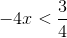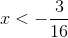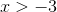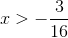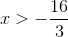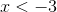Explanation:

To isolate the x-variable, we will need to divide by negative four on both sides.  This is the same as multiplying negative one fourth on both sides.

Since we have a negative in front, we will also need to switch the sign.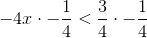Simplify both sides of the equation and switch the sign.The answer is:Example Question #32 : How To Find The Solution To An Inequality With Division

Solve the inequality: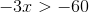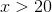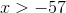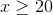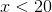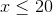Explanation:

Divide by negative three on both sides.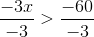Remember that since we are dividing by a negative sign, we will need to switch the sign.

The answer is:Example Question #33 : How To Find The Solution To An Inequality With Division

Solve the inequality: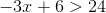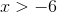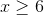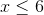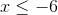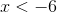Explanation:

Subtract six on both sides.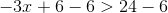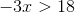Divide by negative three on both sides.  When we divide by a negative number, we will need to switch the direction of the sign.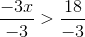Simplify both sides and switch the sign.

The answer is:Example Question #34 : How To Find The Solution To An Inequality With Division

Solve the following inequality: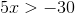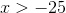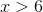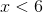Explanation:

Isolate the x-variable by dividing by five on both sides.  There is no need to switch the sign in this scenario.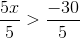Simplify both fraction.

The answer is:Example Question #35 : How To Find The Solution To An Inequality With Division

Solve the inequality: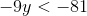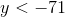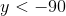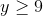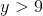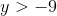Explanation:

To solve this inequality, divide both sides by negative nine.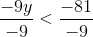Since we are dividing by a negative number, we will need to switch the direction of the sign.

Simplify both sides.

The answer is:Example Question #36 : How To Find The Solution To An Inequality With Division

Solve the following inequality: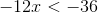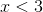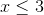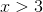Explanation:

Divide by negative twelve on both sides of the inequality.

Dividing by a negative value requires changing the direction of the sign.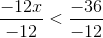Simplify both sides.

The answer is:Example Question #37 : How To Find The Solution To An Inequality With Division

Solve the inequality: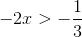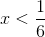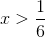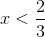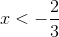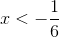Explanation:

Divide both sides by negative two.  We will need to switch the direction of the sign.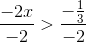Simplify both sides and switch the sign.  Rewrite the complex fraction such that dividing by a negative two is similar to multiplying by negative half.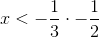Multiply the fractions on the right.

The answer is:Example Question #38 : How To Find The Solution To An Inequality With Division

Solve the inequality: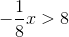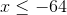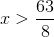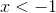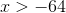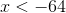Explanation:

Dividing by negative one-eighth is similar to multiplying by negative eight on both sides.  Since we are dividing by a negative number, the sign will switch.Simplify both sides.

The answer is:Dividing by negative one-eighth is similar to multiplying by negative eight on both sides.  Since we are dividing by a negative number, the sign will switch.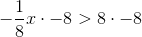Simplify both sides.

The answer is:1 2 4 Next →